Now it’s time for your first project! Let’s start by learning how to use the most basic and commonly used component: the LED. It’s everywhere in life, for lighting, indication, or decoration...

For those coming from the software programming world, you may be familiar with the traditional “hello world” program. In the world of electronics, we have a similar starter project: blinking an LED!

Learning goals

• Understand how the digital output signal works.
• Learn about some new components: diode, LED, resistor.
• Get to know ohm's law and figure out the relations between current, voltage, and resistance.
• Learn about types of circuit connections.
• Start to write code and learn some Swift programming knowledge.

## 🔸Background​

### What is digital output?​

In electronics and telecommunication, electronic signals carry data from one device to another to send and receive all kinds of information. They are always time-varying, which means the voltage changes as time goes on. Different voltages can convey infos and be decoded to a specified message. Depending on the ways the voltage changes, the signals are divided into two types: digital signal and analog signal. You'll take a look at the digital signal in this tutorial.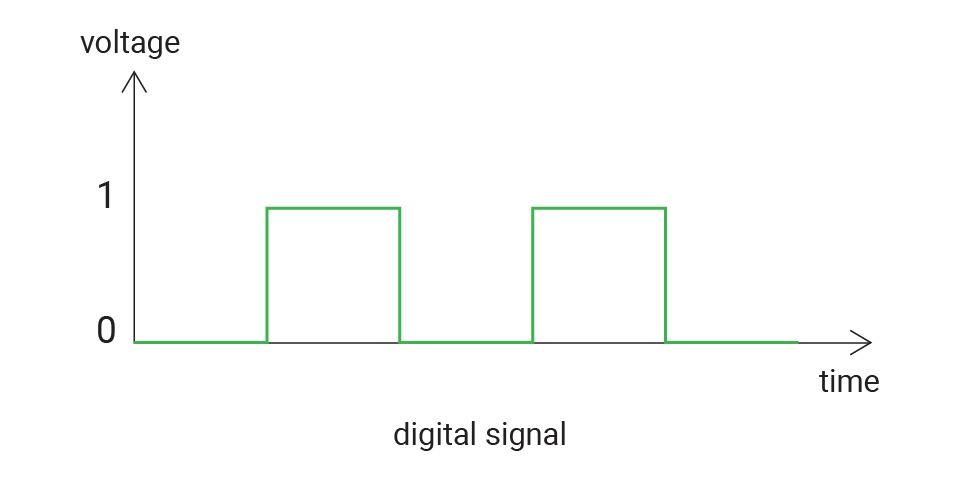In most cases, a digital signal has two states: on or off. It is suitable to work with some components, such LED (which is either turned on or off), the button (which will be only pressed or released) ...

Here are different expressions to represent two voltage states:

Logic 1Logic 0
truefalse
highlow
3.3V0V
##### note

For our board, 3.3V represent true and 0V represent false. Of course, there are many other possibilities, like 5V for true.

GPIO (general-purpose input/output) pins can handle digital output and input signals. You’ll set it as output in your code.

You can use a digital output to control the LED both built onto the board or external LEDs (not included). For the LED module on your kit, when you apply a high signal to the LED, it will turn on, and if you apply a low signal, it will be off.

## 🔸New component​

### Diode​

The diode is a polarized component. It has a positive side (anode) and a negative side (cathode). In the circuit, the current can only flow in a single direction, from anode to cathode. If you connect it in an opposite direction, the current will not be allowed to pass through.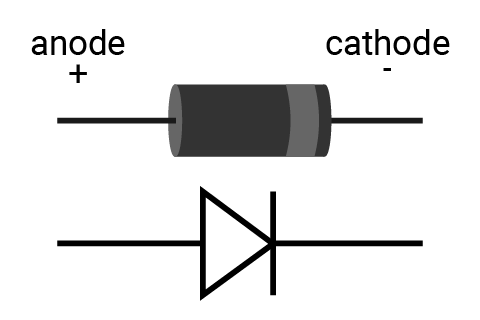Symbol: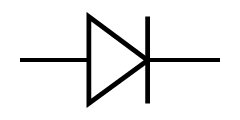### LED​

LED (Light-emitting diode) is a type of diode that will emit light when there is a current. Only when you connect it in the right direction – connect the anode to power and the cathode to ground - is the current allowed to flow, lighting up the LED.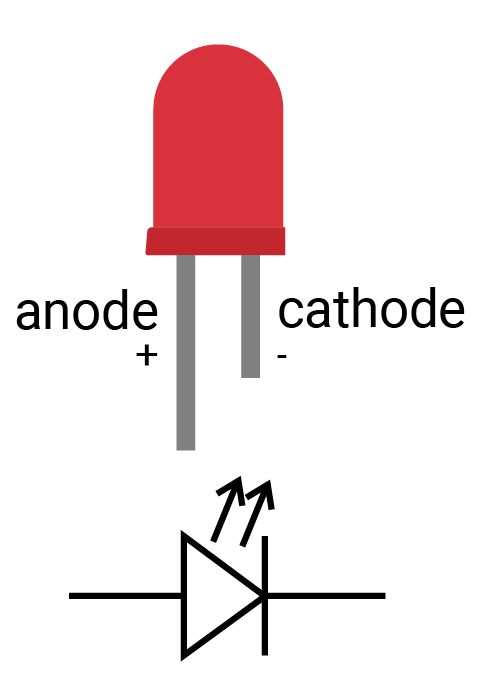Symbol: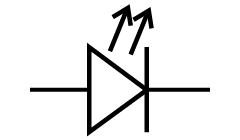##### info

How to identify the two legs of LED?

1. Typically the long leg is positive and the short leg is negative.
2. Alternatively, sometimes you will find a notch on the negative side.

The LED allows a limited range of current, normally no more than 20mA. So you should add a resistor when connecting it to your circuit. Or the LED might burn out when driving too much current.

When you connect the LED in the circuit, there are two cases to control the LED:

• Connect the anode to a digital output pin and cathode to ground. When connected this way, the LED turns on when the pin outputs a high signal. This is how the LED is connected on the Feather board.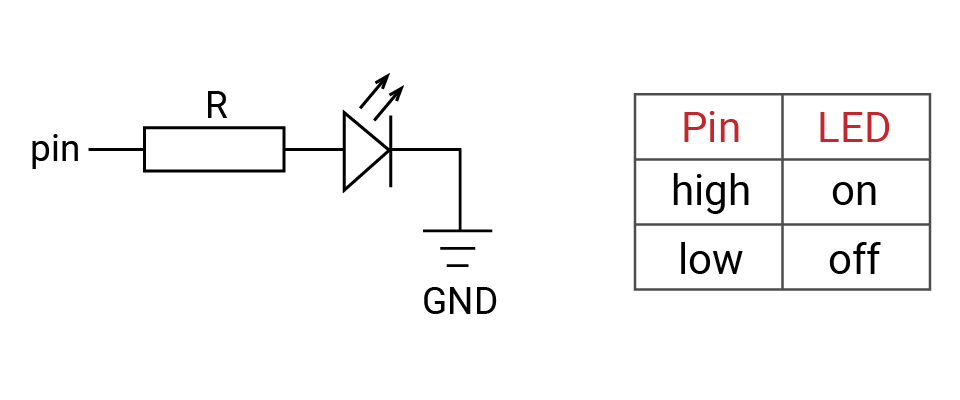Pin is the digital output pin, R is a resistor,is an LED, and GND is ground

• Another method is to connect the anode to a power source and connect the cathode to a digital output pin. When the digital output signal is high, there is no voltage difference between two ends of the LED, but when the digital signal is low, current is allowed to flow, causing the LED to turn on.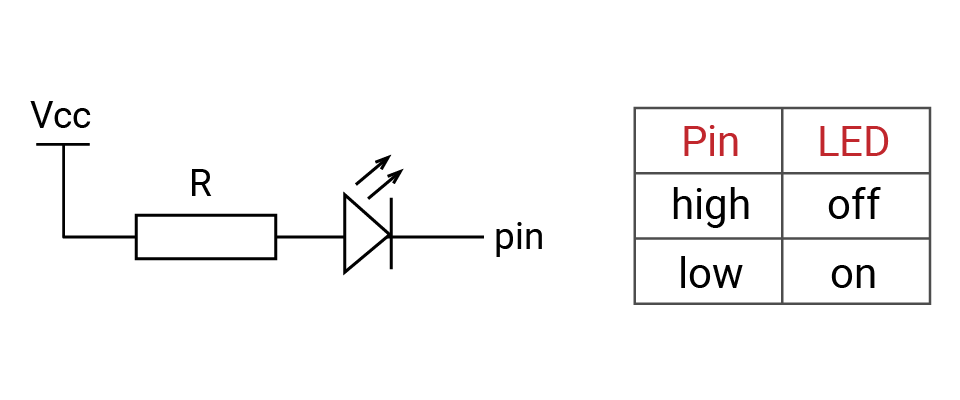Vcc is a power source, R is a resistor,is an LED, and pin is a digital output pin

There are many types of LEDs. The LED on your SwiftIO Circuit Playgrounds is a small variant designed to be convenient for mass production.

### Resistor​

The resistor functions as a current-limiting component which, just as its name suggests, can resist the current in the circuit. It has two legs. You can connect it in either direction as it is not polarized. Its ability to resist the current, called resistance, is measured in ohm (Ω).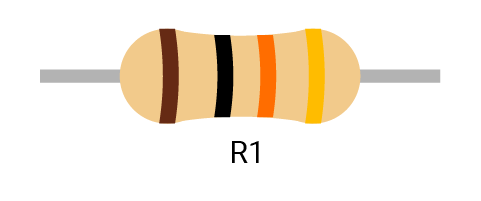Symbol: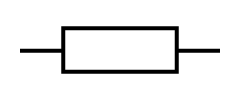(international),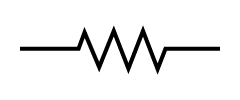(US)

##### info

How can you tell how much resistance a resistor provides?

Each resistor has a specific resistance. Note the colored bands in the diagram. Each band corresponds to a certain number. Here is an online guide and calculator to determine how to total the value of all the bands together.

Challenge

What’s the resistance of the sample resistor R1 pictured above, as well as the resistors R2 and R3 below? See below for the answer!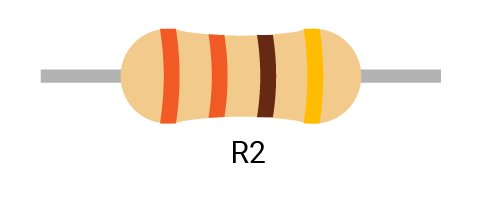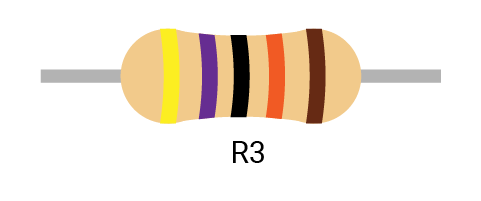• R1: 10KΩ with a tolerance of ± 5%
• R2: 330Ω with a tolerance of ± 5%
• R3: 470KΩ with a tolerance of ± 1%

This kind of resistor is useful primarily when you DIY some stuff. However, the SwiftIO Feather board and the rest of the kit uses surface mount resistors as they are smaller and more suitable for mass production.

## 🔸New concept​

### Ohm’s law​

When starting with electronics, you must get familiar with these three concepts: voltage, current, and resistance:

• Voltage measures potential energy between two points.
• Current describes the rate of flow of electric charges that flow through the circuit.
• And resistance is the capability to resist the flow of current.

An intuitive and common analogy is water pressure in a tank. Imagine a water tank with water inside and an opening at the bottom.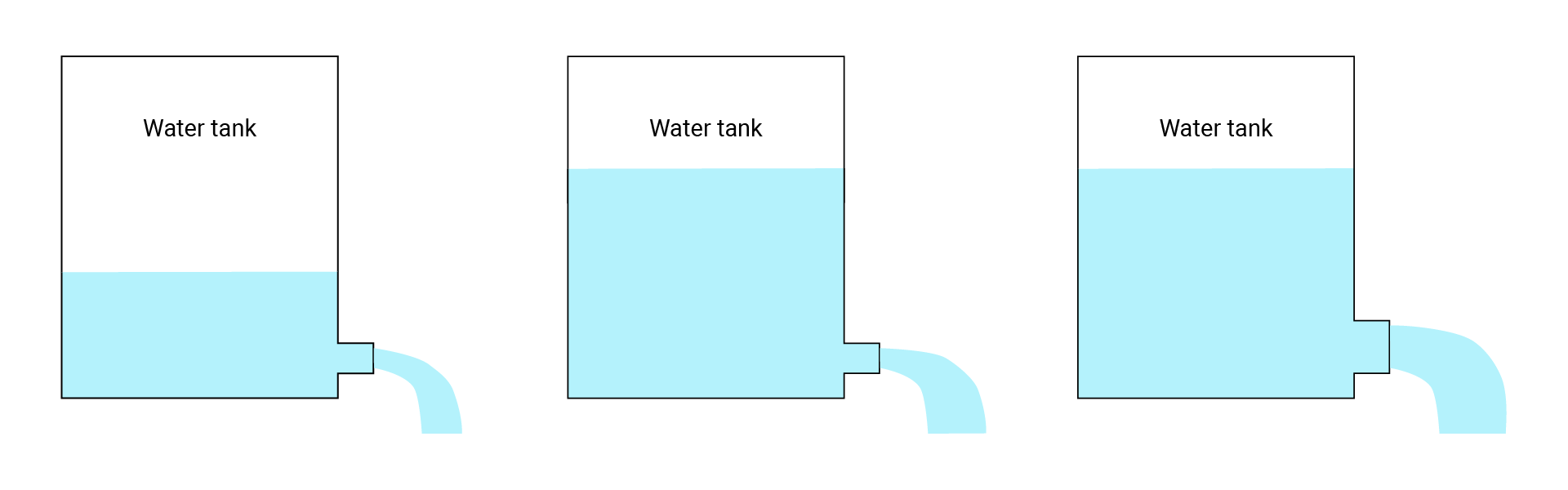In this scenario, the water pressure (water level) is like voltage, the opening is like resistance, and the amount of water spilling out is like current.

• Looking at the first figure, very little water will come out (current) because there isn’t much pressure (voltage) and the opening is small (resistance).
• In the second example, we’ve increased the water level (voltage), but kept the same sized opening (resistance), which results in an increase in the flow of the water (current).
• Finally, in the last one, we’ve also increased the size of the opening (reduced resistance), keeping the water level (voltage) the same, resulting in another increase in flow (current).

Ohm’s law describes how voltage, current and resistance interact with each other and works similar to the water tank above. The formula is:

V = I * R

• V: voltage (unit: volts or V)

• I: current (unit: amps or A)

• R: resistance (unit: ohm or Ω)

Using some simple algebra, we can also put forward the following formulas:

R = V / I

and

I = V / R

As stated previously, all digital pins on the SwiftIO Feather board output a high signal of 3.3V. If the resistance in the circuit is 330Ω, the current would be 0.01A.

##### Exercise

Given an LED with the following characteristics, how many ohm resistor should you use to complete the circuit, using the 3.3V digital out pin source?

• Forward current: 15mA max
• Forward voltage: 3.0V

Here is the equation:

R = (V-Vled) / Iled

• V: supply voltage
• Vled: forward voltage for the LED, that is, voltage drop as the current across the LED.
• Iled: forward current for the LED (usually 10-20mA). It’s the maximum current. If you don’t have the specs about LED, you could normally suppose it to be 20mA.

The resistor needed for the LED is:

R = (3.3 - 3.0) / 0.015 = 20Ω

Btw, the resistance of the LED itself is little so you could ingnore it.

Frequently you will be unable to find a resistor that matches the exact theoretical value. When this happens, you can use a resistor that has a slightly greater resistance.

In general, the resistance calculated is a minimum requirement. You can also choose a resistor with much larger resistance. Doing so will cause the LED’s brightness to change with it. (Greater resistance will cause the LED to be dimmer)

### Serial circuit and parallel circuit​

Serial and parallel circuits are the ways to connect more than two devices in the circuit.

In a serial circuit, devices are connected end-to-end in a chain, like R1 and R2. The current flows through them in one direction from positive to negative. And the current flowing through each device is the same.

In a parallel circuit, the devices share two common nodes, like R3 and R4. Node (a) connects both two devices, so the current could flow through either of them. The voltage between two nodes (a and b) is the same, so the voltages spent on R3 and R4 are the same.In your real situation, the circuit would not be that easy. The serial and parallel circuit would both be used when building the circuit.

Let’s look at an example. You will know better about two circuits.

• In the first circuit, the two lamps are connected in series, so the switch can control both of them. If any of the lamps breaks down, even if the switch is closed, the other lamp will not be lit.
• In the second circuit, the two lamps are connected in parallel, you can control any of them by using the corresponding switch that is connected to it in series: switch1 controls the lamp1, switch2 controls the lamp2. And the two lamps work separately.### Open, closed and short circuit​

In addition to the previously mentioned types of circuits, there are three more you need to know about: open circuit, closed circuit, and short circuit.

• The first figure is a closed circuit. This allows current to flow freely from the positive terminal through a load that consumes electric power, finally returning back to the negative terminal.
• In an open circuit, there is a gap somewhere on the circuit, therefore disallowing any current to flow through it.
• Current tends to flow through the path with lower resistance, if you accidentally connect the positive to the negative terminal of the power supply, the current will flow directly through this path and bypass the other paths with higher resistance. The resistance of wires are so small that you could normally ignore it. This causes a short circuit. When the current reaches sufficiently high levels, this can cause serious damage.### Current safety​

In a complete circuit, the current will always flow from the point of higher voltage (usually power) to the one of lower voltage (usually ground or GND). Consumed energy is turned into light, heat, sound and many other forms.

If you were to connect the power directly to the ground using a wire, this would cause a short circuit. It can (and usually do) cause damage to your circuit and board, and is also very possible to start a fire.

Another warning: if you’re not careful about selecting an appropriately strong resistor to resist the level of current flowing through the circuit, the devices can be burnt and damaged (and additionally cause a fire hazard).

## 🔸Circuit - LED module​

The image below shows how the LED module is connected to the SwiftIO Feather board in a simplified way.

The LED is connected to D19. GND and 3V3 are connected respectively to the corresponding pin on the board.

##### info

On circuit diagrams, the red line is usually for power and the black line for ground.

The circuits are all built when designing the board, so you don’t need to connect any wires. And as mentioned before, the white sockets are used to build the circuit after the board is disassembled.##### note

The circuits above are simplified for your reference.

## 🔸Preparation​

Class

`DigitalOut` - as indicated by its name, this class is used to control digital output, to get high or low voltage.

MethodExplanation
`init(_:mode:value:)`Initialize a digital output pin.
The first parameter needs the pin id which is listed in the Id enumeration. The parameters `mode` and `value` already have a default value: the mode is `pushPull` and the output value is `false`.
`write(_:)`Set the pin to output high or low voltage.
Its parameter is a boolean type: `true` or `false`. `true` corresponds to a high level and `false` corresponds to a low level.
`toggle()`Reverse the digital output between high and low.
`high()`Output high voltage.
`low()`Output low voltage.

Global function

`sleep(ms:)` - Make the microcontroller suspend its work for a certain time, measured in milliseconds.

## 🔸Projects​

In your first try, let’s make the LED blink - on for one second, then off for one second, and repeat it over and over again.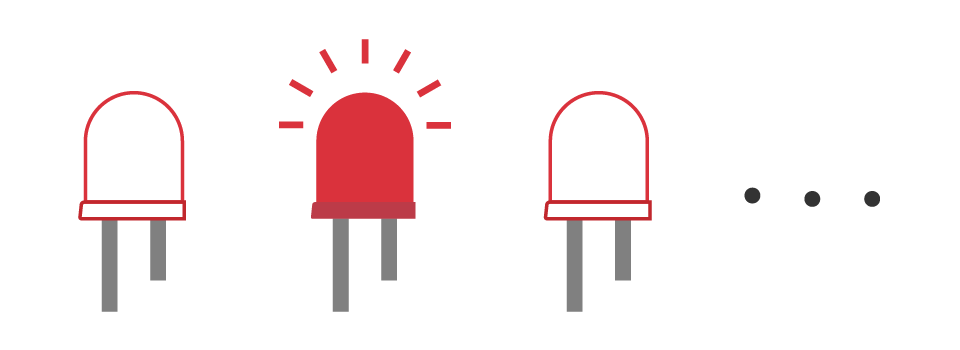Example code

``// First import the SwiftIO and MadBoard libraries into the project to use related functionalities.import SwiftIOimport MadBoard// Initialize the specified pin used for digital output.let led = DigitalOut(Id.D19)// The code in the loop will run over and over again. while true {    //Output high voltage to turn on the LED.       led.write(true)    // Keep the LED on for 1 second.    sleep(ms: 1000)    // Turn off the LED and then keep that state for 1s.    led.write(false)    sleep(ms: 1000)}``

Code analysis

Here are some key statements for this program, make sure you understand them before you start to code.

``// Comment``

This is the comment for the code used to explain how the program works and also for future reference. It always starts with two slashes.

``import SwiftIOimport MadBoard``

These two libraries are necessary for all your projects with the boards. In short, a library contains a predefined collection of code and provides some specified functionalities. You can use the given commands directly without caring about how everything is realized.

`SwiftIO` is used to control input and output. It includes all the necessary commands to talk to your board easily.

`MadBoard` contains the ids of all types boards. The ids for different types of boards may be different since the numbers of pins are not same. Make sure the id used in your code later is correct.

``let led = DigitalOut(Id.D19)``

`let` is the keyword to declare a constant. A constant is like a container whose content will never change. But before using it, you need to declare it in advance. Its name could be whatever you like and it’s better to be descriptive, instead of a random name like abc. If the name of your constant consists of several words, then except the first word, the first letter of the rest words needs to be capitalized, like `ledPin`. This is known as the camel case.

The class, in brief, is more like a mold that you could use to create different examples, known as instances, with similar characteristics. The class `DigitalOut` provides ways to change digital output, so all its instances share the functionalities. The process of creating an instance is called initialization.

The constant `led` is the instance of the `DigitalOut` class. To initialize it,

• The pin id is required. `Id` is an enumeration including the ids of all pins. As for enumeration, enum for short, it could group a set of related values. Just remember that the id needs to be written as `Id.D19`.
• The `mode` of the digital pin is pushPull in most cases and you will know more about it in the future.
• The `value` decides the output state of the pin after it's initialized. By default, it outputs a low level. If you want the pin to output 3.3V by default, the statement should be `let led = DigitalOut(Id.D19, value: true)`.

In this way, the pin D19 would work as a digital output pin and get prepared for the following instructions.

``while true {}``

It’s a dead loop in which the code will run over and over again unless you power off the board. The code block inside the brackets needs to be indented by 4 spaces.

##### note

Sometimes you find nothing that needs to run repeatedly, you could add `sleep` in it to make the board sleep and keep in a known state.

``led.write(true)``

The `led` instance has access to all the instance methods in the `DigitalOut`. `write()` is one of its methods. You’ll use dot syntax to access it: the instance name, followed by a dot, the method in the end. Then you decide the voltage level as its parameter, `true` for high voltage, `false` for low voltage. A value either `true` or `false` is of Boolean type.

``sleep(ms: 1000)``

An instance method needs dot syntax to invoke it, but a global function doesn’t. You can directly call it. The function `sleep(ms:)` has a parameter name `ms` and a parameter (the specified period). During sleep time, the microcontroller would suspend its processes.

##### info

Both methods and functions group a code block, and you could realize the same functionality by calling their name. Their difference is that a method belongs to a class while a function is separately declared.

Why add this statement? The microcontroller executes state change of digital pins extremely quickly. If you just change the output state between high and low, the LED will be on and off so quickly that you cannot notice it. So a short period of time is added here to slow the speed of change. If you want the LED to blink faster, you could reduce the sleep time.

##### info

When using methods or functions, why do some parameters need to add a name and others don't?

Let’s look at the source code below for example:

``func write(_ value: Bool)``

A function parameter has a argument label and a parameter name. The argument label is used when calling a function. While there is an underscore “_” before the parameter name `value`, it means the label can be omitted when invoking the function: `led.write(true)`.

``func sleep(ms: Int)``

In this case, `ms` serves as the argument label by default, so it's necessary: `sleep(ms: 1000)`.

### 2. LED morse code​

Example code

Have you heard of Morse code? It encodes characters into a sequence of dashes and dots to send messages. To reproduce it, you could use long flash and short flash respectively. In morse code, s is represented by three dots, o is represented by three dashes. So the SOS signal needs three short flashes, three long flashes, and then three short flashes again.

``// Import the libraries to use all their functionalities.import SwiftIOimport MadBoard// Initialize the digital output pin.let led = DigitalOut(Id.D19)// Define the LED states to represent the letter s and o.let sSignal = [false, false, false]let oSignal = [true, true ,true]// Set the LED blink rate according to the values in the array.func send(_ values: [Bool], to light: DigitalOut) {    // The duration of slow flash and quick flash.    let long = 1000    let short = 500    // Iterate all the values in the array.     // If the value is true, the LED will be on for 1s, which is a slow flash.    // And if it’s false, the LED will be on for 0.5s, which is a quick flash.    for value in values {        light.high()        if value {            sleep(ms: long)        } else {            sleep(ms: short)        }        light.low()        sleep(ms: short)    }}// Blink the LED.// At first, the LED starts 3 fast blink to represent s, then 3 slow blink to represent o, and 3 fast blink again.// Wait 1s before repeating again.while true {    send(sSignal, to: led)    send(oSignal, to: led)    send(sSignal, to: led)    sleep(ms: 1000)}``

Code analysis

``let sSignal = [false, false, false]let oSignal = [true, true, true]``

Here, two arrays are used to store the info of two letters. Since there are only two states: fast or slow flash, you could use boolean value to represent two states. `false` corresponds to quick flash.

An array stores a series of ordered values of the same type in a pair of square brackets. The values above are all boolean values.

``func send(_ values: [Bool], to light: DigitalOut) {    ...}``

You will create a function to finish blinks for a single letter. It needs two parameters: the first is an array of boolean values that stores the info for a letter; the second is the digital pin that the LED is connected to.

This function is to make your code more organized and clear. Of course, you can use other ways of abstraction.

##### info

Usually, it's better not to use the variable or constants that are declared out of the function itself. All stuff needed is passed in as its parameters. As you invoke the function, you will then tell which pin is going to be used and what the values are. Thus you could use this piece of code in other project without modifying the code. This practice would be really helpful as you work on great projects in the future.

``let long = 1000let short = 500``

Set the duration of LED on-time. The values are stored in two constants so it would be clearer as you use them later.

``for value in values {    ...}``

This is a for-in loop. It has two keywords: `for` and `in`. It’s used to repeat similar operations for each element and usually used with arrays. The code inside the curly brackets would repeat several times to iterate all elements in the array.

`value` represents the elements in the array `values`. It doesn’t matter if you use `value` or a, b, c to name it. But it’s better to use a descriptive name.

``if condition {    task1} else {    task2}``

This is a conditional statement. The if-else statement makes it possible to do different tasks according to the condition. The condition is always a boolean expression that will return either true or false. And it will use some comparison operators to evaluate the value as follows:

• Equal to: `a == b`
• Not equal to: `a != b`
• Greater than: `a > b`
• Less than: `a < b`
• Greater than or equal to: `a >= b`
• Less than or equal to: `a <= b`

In the code above, the `value` is judged to know how long the LED should be on.
``light.high()light.low()``
Set high or low voltage. This is similar to the method `write()`, but it’s more straightforward. The statement `led.write(true)` of course works.